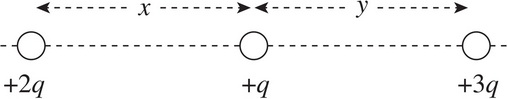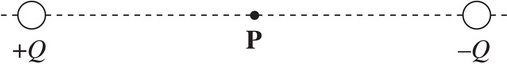# AP Physics 1: Electric Forces and Fields Multiple-Choice Practice Questions

### Test Information9 questions18 minutes

1. If the distance between two positive point charges is tripled, then the strength of the electrostatic repulsion between them will decrease by a factor of

2. Two 1 kg spheres each carry a charge of magnitude 1 C. How does FE, the strength of the electric force between the spheres, compare to FG, the strength of their gravitational attraction?

3. The figure below shows three point charges, all positive. If the net electric force on the center charge is zero, what is the value of y/x ?4. The figure above shows two point charges, +Q and -Q. If the negative charge were absent, the electric field at Point P due to +Q would have strength E. With -Q in place, what is the strength of the total electric field at P, which lies at the midpoint of the line segment joining the charges?

5. A sphere of charge +Q is fixed in position. A smaller sphere of charge +q is placed near the larger sphere and released from rest. The small sphere will move away from the large sphere with

6. An object of charge +q feels an electric force FE when placed at a particular location in an electric field, E. Therefore, if an object of charge -2q were placed at the same location where the first charge was, it would feel an electric force of

7. A charge of -3Q is transferred to a solid metal sphere of radius r. Where will this excess charge reside?

8. How far apart are two charges (q1 = 8 × 10-6 C and q2 = 6 × 10-6 C) if the electric force exerted by the charges on each other has a magnitude of 2.7 × 10-2 N ?

9. Two charges (q1 and q2) are separated by a distance r. If the ratio of FG/FE is equal to 9.0 × 1043, what is the new ratio if the distance between the two charges is now 3r ?